# 2. Week 02 - Validation¶

Note

This is a written version of Lecture #2, Iteration #2.

It covers low-level, untyped on-chain validation scripts and high-level, typed on-chain validation scripts.

The code in this lecture uses the Plutus commit 81ba78edb1d634a13371397d8c8b19829345ce0d

## 2.1. Before We Start¶

Let’s talk about an important point that was brought up by one of the pioneers following lecture #1.

You will recall in the auction example, we created three endpoints - `start`, `bid` and `close`. For `close` there were two scenarios. If there was a high-enough bid, the token goes to the highest bidder. If there was not a high-enough bid, the token goes back to the seller.

What would happen if the `close` endpoint wasn’t there? Could the money be locked forever in the contract?

This is a really important point, because what you have to realise is that that UTxOs on the blockchain are just data, they are absolutely passive. In order for anything to happen there must be a transaction. In order to make progress and to change the state of the blockchain, there must be a new transaction submitted that consumes various UTxOs and produces new UTxOs.

Only new transactions change the state. A UTxO will never spring into action by itself and do something. You can’t have a smart contract that sits on the blockchain and the, at some point, suddenly performs an action.

So, we really need the `close` endpoint if we want the auction to be settled. In our case, the endpoint was manually triggered. You could write a contract that runs in the wallet that would automatically generate the `close` transaction - it is possible to write quite sophisticated off-chain code.

However, from the point-of-view of the blockchain, it is always an external trigger that does something. Nothing happens if it is not externally triggered.

So, if there were no `close` endpoint, or the `close` endpoint never got triggered, the funds would remain sitting at the script address forever.

## 2.2. Introduction¶

We saw in the first lecture that there are two sides to a smart contract - an on-chain part and an off-chain part.

The on-chain part is about validation. It allows nodes to validate a given transaction and whether it is allowed to consume a given UTxO.

The off-chain part lives in the user’s wallet. It constructs and submits suitable transactions.

Both are important topics. We have to master both in order to write smart contracts, but for now we will concentrate on the on-chain part.

Let’s recall the Extended UTxO model where the idea is that we introduce a new type of address.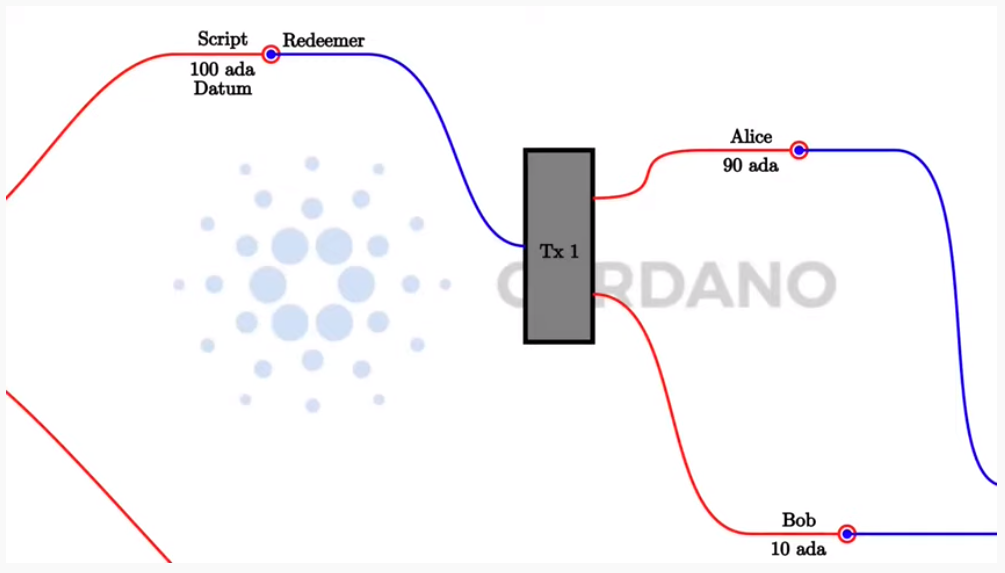In the simple UTxO model are so-called public key addresses, where the address is given by the hash of the public key. If a UTxO sits at such a public key address, then a transaction can consume that UTxO as an input if the signature belonging to that public key is included in the transaction.

What the (E)UTxO model does is extend this by adding script addresses that can run arbitrary logic.

When a transaction wants to consume a UTxO sitting at a script address is validated by a node, the node will run the script and then, depending on the result of the script, decide whether the transaction is valid or not.

And recall that two were three more additions:

1. Instead of just having signatures on transactions, we have so-called Redeemers - arbitrary pieces of data.

2. On the UTxO output side, we have an additional arbitrary piece of data called Datum, which you can think of as a little piece of state that sits on the UTxO.

Finally, we have the context. There are various choices of what this context can be. It can be very restrictive, consisting just of the Redeemer (as in Bitcoin), or very global, consisting of the whole state of the blockchain (as in Ethereum). In Cardano, it is the transaction that is being validated, including all its inputs and outputs.

So, there are three pieces of data that a Plutus script gets. The Datum, sitting at the UTxO, the redeemer, coming from the input and the validation, and the context, consisting of the transaction being validated and its inputs and outputs.

In a concrete implementation like Plutus, these pieces of information need to be represented by a concrete data type - a Haskell data type. As it happens, the choice was made to use the same data type for all three of them. At least at the low-level implementation.

We will look at that first, but in real life nobody would actually use this low-level approach. There are more convenient ways to use more suitable data types for these things, and we will come to that later in this lecture.

## 2.3. PlutusTx.Data¶

As mentioned, the datum, redeemer and context share a data type.

That data type is defined in the package `plutus-core`, in the module `PlutusCore.Data`.

It is called, simply, `Data`.

```data Data =
Constr Integer [Data]
| Map [(Data, Data)]
| List [Data]
| I Integer
| B BS.ByteString
deriving stock (Show, Eq, Ord, Generic)
deriving anyclass (NFData)
```

It has five constructors.

• `Constr` takes an Integer and, recursively, a list of `Data`

• `Map` takes a list of pairs of Data. You can think of this as a lookup table of key-value pairs where both the key and the value are of type `Data`

• `List` takes a list of `Data`

• `I` takes a single Integer

• `B` takes a Bytestring

For those familiar with the JSON format, this is very similar. The constructors are not exactly the same, but, like JSON, you can represent numbers, strings, lists of data and key-value pairs. It can represent arbitrary data, which makes it very suitable for our purpose.

We can also explore this type in the REPL.

Run the following from the plutus-pioneers-program repository. You may need to start a nix-shell from the Plutus repository before changing into the `week02` directory.

```cd code/week02
cabal repl
```

From with the REPL, we need to import `PlutusTx` so that we have access to the `Data` type. `Data` is not defined in `PlutusTx`, but it gets re-exported from there.

```import PlutusTx
```

We can now get some information about `Data`.

```:i Data
```

This will give information about the type Data.

```Prelude Week02.Burn> import PlutusTx
Prelude PlutusTx Week02.Burn> :i Data
type Data :: *
data Data
= Constr Integer [Data]
| Map [(Data, Data)]
| List [Data]
| I Integer
| B bytestring-0.10.12.0:Data.ByteString.Internal.ByteString
-- Defined in ‘plutus-core-0.1.0.0:PlutusCore.Data’
instance Eq Data
-- Defined in ‘plutus-core-0.1.0.0:PlutusCore.Data’
instance Ord Data
-- Defined in ‘plutus-core-0.1.0.0:PlutusCore.Data’
instance Show Data
-- Defined in ‘plutus-core-0.1.0.0:PlutusCore.Data’
instance IsData Data -- Defined in ‘PlutusTx.IsData.Class’
```

Now we can play with it. We can use the `I` constructor to create a value of type `Data`.

```Prelude PlutusTx.Data Week02.Burn> I 42
I 42
```

We can ask for its type, and confirm that it is indeed of type `Data`:

```Prelude PlutusTx.Data Week02.Burn> :t I 42
I 42 :: Data
```

The easiest way to create a value of type `Data` using the `B` constructor is to use the GHC Extension `OverloadedStrings`. This allows literal strings to be used in place of string-like data types and the compiler will interpret them as their intended type.

```Prelude PlutusTx.Data Week02.Burn> :set -XOverloadedStrings
Prelude PlutusTx.Data Week02.Burn> :t B "Haskell"
```

We can also use more complicated constructors, like `Map` and `List`:

```Prelude PlutusTx.Data Week02.Burn> :t Map [(I 42, B "Haskell"), (List [I 0], I 1000)]
Map [(I 42, B "Haskell"), (List [I 0], I 1000)] :: Data
```

## 2.4. Plutus Validator¶

Now we are ready to implement our very first validator.

### 2.4.1. Example 1 - The Gift Contract¶

#### 2.4.1.1. The Code¶

We start the script by copy pasting a list of GHC language extensions, plus some dependency imports from the example we used in the last lecture.

```{-# LANGUAGE DataKinds           #-}
{-# LANGUAGE FlexibleContexts    #-}
...

import           Data.Map            as Map
...
import           Text.Printf         (printf)
```

Then, we write the validator. Ultimately, the validator will be a script, living on the blockchain in Plutus Core, which is a lower-level language based on the lambda calculus. But, we don’t have to write Plutus Core. We can write Haskell and we will see later how we convert that Haskell into Plutus Core script.

So, we write a Haskell function that represents our validator. As we know, a validator is a script that takes three pieces of input - the datum, the redeemer and the context, respectively, which, at the lowest level are represented by the `Data` data type.

```mkValidator :: Data -> Data -> Data -> ()
```

Somewhat surprisingly, the result of the function is `()`. This is the Haskell `Unit` type, similar to `void` in some other languages, like C or C# or Java - it’s the type that carries no information.

`Unit` is a built-in type in Haskell and it has just one value, which is written in the same way as the type itself, as we can see from the REPL.

```Prelude Week02.Gift> ()
()
() :: ()
```

A function with a return type of `()` is quite unusual in Haskell. In more mainstream languages, it is quite common for functions or procedures to return no value. In these situations, the functions are only important for their side-effects, such as a Java function that prints something to the console.

But Haskell is a pure language. If you want side-effects, this will be shown by the type system. For example if the mkValidator were to perform any IO, it would have a type signature of:

```mkValidator :: Data -> Data -> Data -> IO ()
```

This would indicate a function that performs IO side-effects but has no interesting return value.

But, as we know that the real `mkValidator` function performs no side-effects and returns no value, there is really nothing useful that it can do.

However, there is something that the function can do as well as returning `()`, namely it can throw an exception or have an error. And that’s what Plutus uses.

The idea is that if the `mkValidator` function does not run into an error or throw an exception, then validation succeeds. If it throws an error then validation fails and the transaction is rejected.

Let’s write the simplest validator that we can.

```mkValidator :: Data -> Data -> Data -> ()
mkValidator _ _ _ = ()
```

The first argument is the datum, the second argument is the redeemer and the third argument is the context. The most simple thing we can do is to completely ignore all three arguments and immediately return `()`.

What this means is that the script address that corresponds to this validator doesn’t care about the datum, it doesn’t care about the redeemer, and it doesn’t care about the Context. It will always succeed, and this means that any transaction can consume the script at this address as an input. It does not matter what datum exists for a UTxO at this script address, it doesn’t matter which redeemer is used for the transaction and it doesn’t matter what structure the transaction has.

If you send any funds to this script address, anybody can immediately take it.

This function is not yet Plutus code, it is just a Haskell function. In order to turn it into a Plutus script, we need to compile it.

The result of our compilation to Plutus will be of type `Validator`. Below the function in `Gift.hs`, we add the following code.

```validator :: Validator
validator = mkValidatorScript \$\$(PlutusTx.compile [|| mkValidator ||])
```

The `mkValidatorScript` function takes the type `CompiledCode (Data -> Data -> Data -> ()) -> Validator`. In order to create this type, we must compile the `mkValidator` script using something called Template Haskell.

Template Haskell is an advanced feature of Haskell that solves a similar problem as macro systems in other languages. A macro being something that gets expanded at compile time.

So, with this code

```\$\$(PlutusTx.compile [|| mkValidator ||])
```

We are asking the compiler to write the code for the `validator` function at compile time based on our `mkValidator` function, and then proceed with the normal compilation.

You do not need to understand very much about Template Haskell to write Plutus as it is always the same pattern. Once you have seen a couple of examples, you can more or less just copy and paste.

Template Haskell expects all the code to be available within the Oxford Brackets - `[| |]`.

With more complicated validators you will likely be relying on multiple helper functions, and you do not want to have to add them within the Oxford Brackets. To avoid this, there is one thing we need to do to the `mkValidator` function, and that is to make it inlinable by adding the `INLINABLE` pragma.

```{-# INLINABLE mkValidator #-}
mkValidator :: Data -> Data -> Data -> ()
mkValidator _ _ _ = ()
```

You will see this often in Plutus scripts, and it is usually an indication that a function is meant to be used within a validation script. All the functions on which the validator depends must be inlinable.

Let’s go back to the REPL and take a look at the validator.

```:l src/Week02/Gift.hs
Validator { <script> }
```

We can ask for information about `Validator`.

```Prelude PlutusTx Ledger.Scripts Week02.Gift> :i Validator
type Validator :: *
newtype Validator = Validator {getValidator :: Script}
-- Defined in ‘plutus-ledger-api-0.1.0.0:Plutus.V1.Ledger.Scripts’
instance Eq Validator
-- Defined in ‘plutus-ledger-api-0.1.0.0:Plutus.V1.Ledger.Scripts’
instance Ord Validator
-- Defined in ‘plutus-ledger-api-0.1.0.0:Plutus.V1.Ledger.Scripts’
instance Show Validator
-- Defined in ‘plutus-ledger-api-0.1.0.0:Plutus.V1.Ledger.Scripts’
```

We see that it is a wrapper around `getValidator`

```Prelude PlutusTx Ledger.Scripts Week02.Gift> getValidator validator
<Script>
```

We can then get some information about `Script`

```Prelude PlutusTx Ledger.Scripts Week02.Gift> :i Script
type Script :: *
newtype Script
= Script {unScript :: plutus-core-0.1.0.0:UntypedPlutusCore.Core.Type.Program
plutus-core-0.1.0.0:PlutusCore.DeBruijn.Internal.DeBruijn
plutus-core-0.1.0.0:PlutusCore.Default.Universe.DefaultUni
plutus-core-0.1.0.0:PlutusCore.Default.Builtins.DefaultFun
()}
-- Defined in ‘plutus-ledger-api-0.1.0.0:Plutus.V1.Ledger.Scripts’
instance Eq Script
-- Defined in ‘plutus-ledger-api-0.1.0.0:Plutus.V1.Ledger.Scripts’
instance Ord Script
-- Defined in ‘plutus-ledger-api-0.1.0.0:Plutus.V1.Ledger.Scripts’
instance Show Script
-- Defined in ‘plutus-ledger-api-0.1.0.0:Plutus.V1.Ledger.Scripts’
```

And here we see that we have an `unScript` function, which we can run

```Prelude PlutusTx Ledger.Scripts Week02.Gift> unScript \$ getValidator validator
Program () (Version () 1 0 0) (Apply () (Apply () (LamAbs () (DeBruijn {dbnIndex = 0}) (LamAbs () (DeBruijn {dbnIndex = 0}) (Apply () (Apply () (Apply () (LamAbs () (DeBruijn {dbnIndex = 0}) (LamAbs () (DeBruijn {dbnIndex = 0}) (LamAbs () (DeBruijn {dbnIndex = 0}) (Apply () (Apply () (Apply () (Apply () (Apply () (Apply () (LamAbs () (DeBruijn {dbnIndex = 0}) (LamAbs () (DeBruijn {dbnIndex = 0}) (LamAbs () (DeBruijn {dbnIndex = 0}) (LamAbs () (DeBruijn {dbnIndex = 0}) (LamAbs () (DeBruijn {dbnIndex = 0}) (LamAbs () (DeBruijn {dbnIndex = 0}) (Apply () (Apply () (LamAbs () (DeBruijn {dbnIndex = 0}) (LamAbs () (DeBruijn {dbnIndex = 0}) (Apply () (LamAbs () (DeBruijn {dbnIndex = 0}) (Var () (DeBruijn {dbnIndex = 1}))) (LamAbs () (DeBruijn {dbnIndex = 0}) (LamAbs () (DeBruijn {dbnIndex = 0}) (LamAbs () (DeBruijn {dbnIndex = 0}) (Var () (DeBruijn {dbnIndex = 5})))))))) (Delay () (LamAbs () (DeBruijn {dbnIndex = 0}) (Var () (DeBruijn {dbnIndex = 1}))))) (LamAbs () (DeBruijn {dbnIndex = 0}) (Var () (DeBruijn {dbnIndex = 1})))))))))) (LamAbs () (DeBruijn {dbnIndex = 0}) (Delay () (LamAbs () (DeBruijn {dbnIndex = 0}) (LamAbs () (DeBruijn {dbnIndex = 0}) (LamAbs () (DeBruijn {dbnIndex = 0}) (LamAbs () (DeBruijn {dbnIndex = 0}) (LamAbs () (DeBruijn {dbnIndex = 0}) (Apply () (Var () (DeBruijn {dbnIndex = 5})) (Var () (DeBruijn {dbnIndex = 6}))))))))))) (LamAbs () (DeBruijn {dbnIndex = 0}) (LamAbs () (DeBruijn {dbnIndex = 0}) (Delay () (LamAbs () (DeBruijn {dbnIndex = 0}) (LamAbs () (DeBruijn {dbnIndex = 0}) (LamAbs () (DeBruijn {dbnIndex = 0}) (LamAbs () (DeBruijn {dbnIndex = 0}) (LamAbs () (DeBruijn {dbnIndex = 0}) (Apply () (Apply () (Var () (DeBruijn {dbnIndex = 4})) (Var () (DeBruijn {dbnIndex = 7}))) (Var () (DeBruijn {dbnIndex = 6})))))))))))) (LamAbs () (DeBruijn {dbnIndex = 0}) (Delay () (LamAbs () (DeBruijn {dbnIndex = 0}) (LamAbs () (DeBruijn {dbnIndex = 0}) (LamAbs () (DeBruijn {dbnIndex = 0}) (LamAbs () (DeBruijn {dbnIndex = 0}) (LamAbs () (DeBruijn {dbnIndex = 0}) (Apply () (Var () (DeBruijn {dbnIndex = 3})) (Var () (DeBruijn {dbnIndex = 6}))))))))))) (LamAbs () (DeBruijn {dbnIndex = 0}) (Delay () (LamAbs () (DeBruijn {dbnIndex = 0}) (LamAbs () (DeBruijn {dbnIndex = 0}) (LamAbs () (DeBruijn {dbnIndex = 0}) (LamAbs () (DeBruijn {dbnIndex = 0}) (LamAbs () (DeBruijn {dbnIndex = 0}) (Apply () (Var () (DeBruijn {dbnIndex = 2})) (Var () (DeBruijn {dbnIndex = 6}))))))))))) (LamAbs () (DeBruijn {dbnIndex = 0}) (Delay () (LamAbs () (DeBruijn {dbnIndex = 0}) (LamAbs () (DeBruijn {dbnIndex = 0}) (LamAbs () (DeBruijn {dbnIndex = 0}) (LamAbs () (DeBruijn {dbnIndex = 0}) (LamAbs () (DeBruijn {dbnIndex = 0}) (Apply () (Var () (DeBruijn {dbnIndex = 1})) (Var () (DeBruijn {dbnIndex = 6}))))))))))) (LamAbs () (DeBruijn {dbnIndex = 0}) (Var () (DeBruijn {dbnIndex = 1}))))))) (Delay () (Delay () (LamAbs () (DeBruijn {dbnIndex = 0}) (LamAbs () (DeBruijn {dbnIndex = 0}) (Var () (DeBruijn {dbnIndex = 2}))))))) (Delay () (LamAbs () (DeBruijn {dbnIndex = 0}) (LamAbs () (DeBruijn {dbnIndex = 0}) (Delay () (LamAbs () (DeBruijn {dbnIndex = 0}) (LamAbs () (DeBruijn {dbnIndex = 0}) (Apply () (Apply () (Var () (DeBruijn {dbnIndex = 1})) (Var () (DeBruijn {dbnIndex = 4}))) (Var () (DeBruijn {dbnIndex = 3})))))))))) (Delay () (LamAbs () (DeBruijn {dbnIndex = 0}) (Var () (DeBruijn {dbnIndex = 1}))))))) (Delay () (Delay () (LamAbs () (DeBruijn {dbnIndex = 0}) (LamAbs () (DeBruijn {dbnIndex = 0}) (Delay () (LamAbs () (DeBruijn {dbnIndex = 0}) (Apply () (Apply () (Var () (DeBruijn {dbnIndex = 1})) (Var () (DeBruijn {dbnIndex = 3}))) (Var () (DeBruijn {dbnIndex = 2})))))))))) (Delay () (Delay () (LamAbs () (DeBruijn {dbnIndex = 0}) (Var () (DeBruijn {dbnIndex = 1}))))))
```

And here you can see an honest-to-goodness representation of the Plutus Core script for the validator.

Back to the code.

Now we have our first validator, there are two more types that we can define.

One is the `ValidatorHash`, which, as the name suggests is the hash of the validator.

```valHash :: Ledger.ValidatorHash
valHash = Scripts.validatorHash validator
```

And, we can also turn the validator into a script address, which is the script’s address on the blockchain.

```scrAddress :: Ledger.Address
```

Now we have a script address represented as `scrAddress`.

We can look at these two results in the REPL

```Prelude PlutusTx Ledger.Scripts Week02.Gift> valHash

```

With the exception of the `mkValidator` function logic (in our case, one line), the rest of the code we have written so far is boilerplate and will be very similar for all Plutus scripts.

In order to actually try this script, we need wallet code. The focus of this lecture is validation and not wallet code, but briefly, here is the rest of the code.

Two endpoints are defined. Endpoints are ways for a user to trigger something with input parameters.

The `give` endpoint will take an `Integer` argument to specify the number of lovelace that will be deposited to the contract.

The `grab` endpoint will take no argument and will simply look for UTxOs at this script address and consume them.

```type GiftSchema =
Endpoint "give" Integer
.\/ Endpoint "grab" ()
```

The `give` endpoint uses the helper function `mustPayToOtherScript` which takes the `valHash` of the recipient script and a `Datum` that, in this example, is completely ignored. It uses the `Datum` constructor to turn a `Data` into a `Datum`. In this case the `Data` is created using the `Constr` constructor taking a 0 and an empty list.

Finally the amount to send to the address is specified using the helper function `Ada.lovelaceValueOf`.

The transaction is then submitted, the script waits for it to be confirmed and then prints a log message.

```give :: AsContractError e => Integer -> Contract w s e ()
give amount = do
let tx = mustPayToOtherScript valHash (Datum \$ Constr 0 []) \$ Ada.lovelaceValueOf amount
ledgerTx <- submitTx tx
void \$ awaitTxConfirmed \$ txId ledgerTx
```

The `grab` endpoint is a little bit more complicated.

We use `utxoAt` with our new script address `scrAddress` to lookup all the UTxOs sitting at that address. We then need lookups, which will be used by the wallet to construct the transaction. Here, we tell the wallet where to find all the UTxOs, and we inform it about the validator. Remember, if you want to consume a UTxO sitting at a script address, then the spending transaction needs to provide the validator code, whereas the transaction that produces the UTxO only needs to provide the hash.

We then define the transaction by using `mustSpendScriptOutput` for each UTxO found. This is saying that every UTxO sitting at this script address must be spent by the transaction we are constructing.

We also pass a redeemer which is completely ignored in our example, so we can put anything there - in this case a redeemer created using the `I` constructor of type `Data` with a value of `17`.

Again, we submit, wait for confirmation, and then write a log message.

```grab :: forall w s e. AsContractError e => Contract w s e ()
grab = do
let orefs   = fst <\$> Map.toList utxos
lookups  = Constraints.unspentOutputs utxos      <>
Constraints.otherScript validator
tx :: TxConstraints Void Void
tx       = mconcat [mustSpendScriptOutput oref \$ Redeemer \$ I 17 | oref <- orefs]
ledgerTx <- submitTxConstraintsWith @Void lookups tx
void \$ awaitTxConfirmed \$ txId ledgerTx
```

Finally, we put it all together in the `endpoints` function. This is boilerplate code that is telling the wallet to give the option of certain endpoints to the user and then, once one has been selected, to recurse and continue to offer the same options again and again. In the case of `give` the user will be required to provide the `Integer` argument.

```endpoints :: Contract () GiftSchema Text ()
endpoints = (give' `select` grab') >> endpoints
where
give' = endpoint @"give" >>= give
grab' = endpoint @"grab" >>  grab
```

Then we have a little piece of boilerplate.

```mkSchemaDefinitions ''GiftSchema
```

And then some code that is used only by the Plutus Playground which allows us to specify additional tokens that can be used for testing.

```mkKnownCurrencies []
```

#### 2.4.1.2. Testing¶

We will now test the `Gift` script in the playground.

Copy the `Gift` script into the playground, then compile the script in the playground and press the `Simulate` button.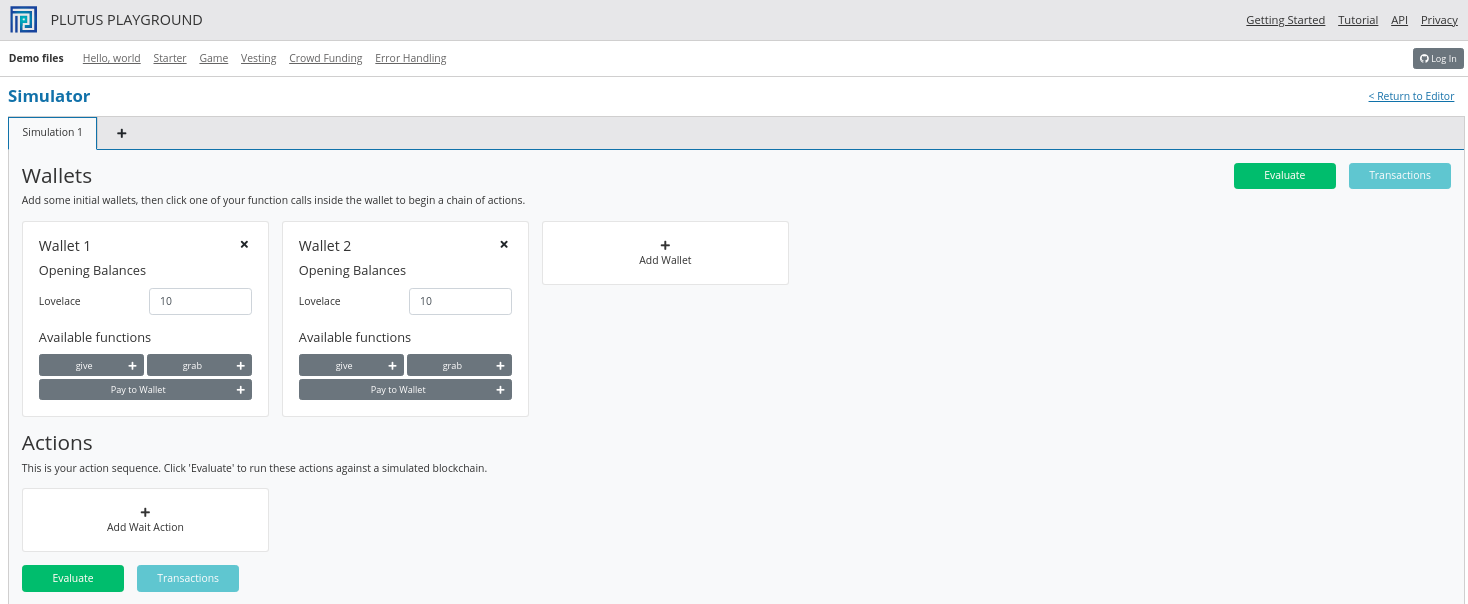And let’s add a third wallet and give all the wallets 10 Ada (10 million lovelace).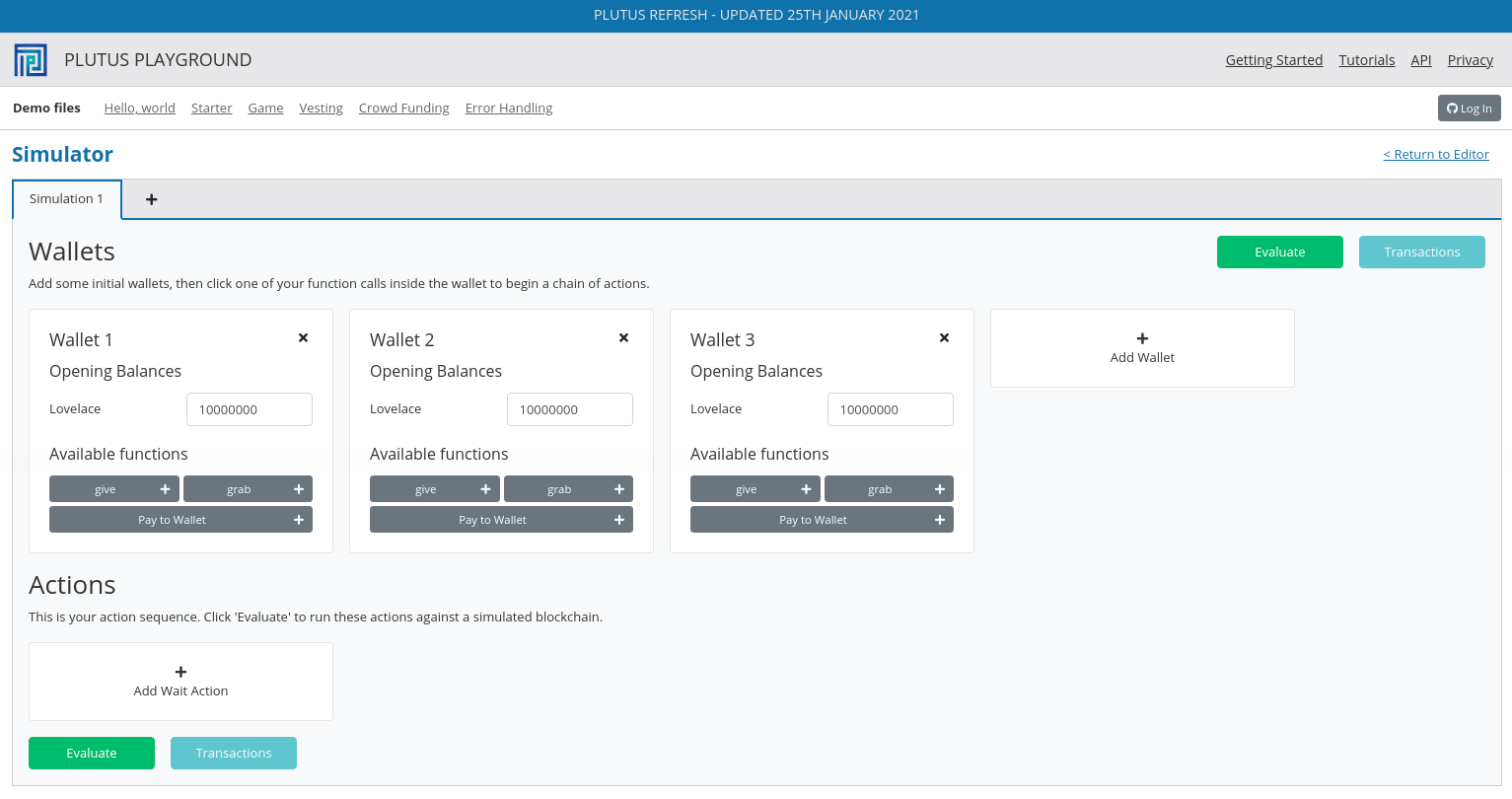We will create a scenario where wallets 1 and 2 give lovelace, and wallet 3 grabs all of it.

You will see that the playground has rendered UI buttons for the two endpoints `give` and `grab`. Use the `give` endpoint for to make wallet 1 give 4 Ada and to make wallet 2 give 6 Ada. Then add a wait action to wait for 1 block, and then use to `grab` endpoint to make wallet 3 grab the funds. Then add another wait action to wait for 1 block.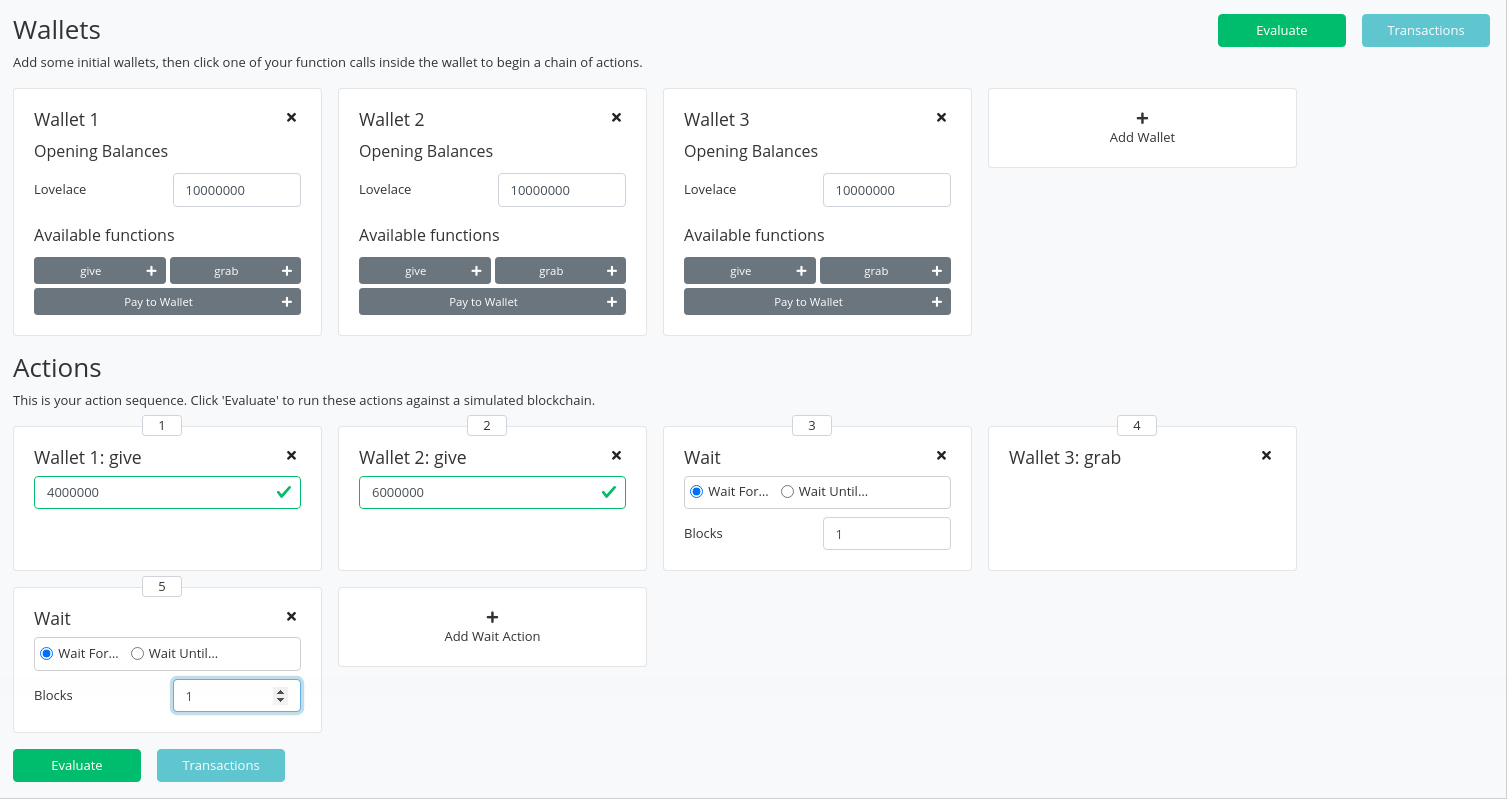And now click `Evaluate`. We see that there have been four transactions.

The first transaction is, as always, the genesis transaction that distributes the initial funds to the wallets.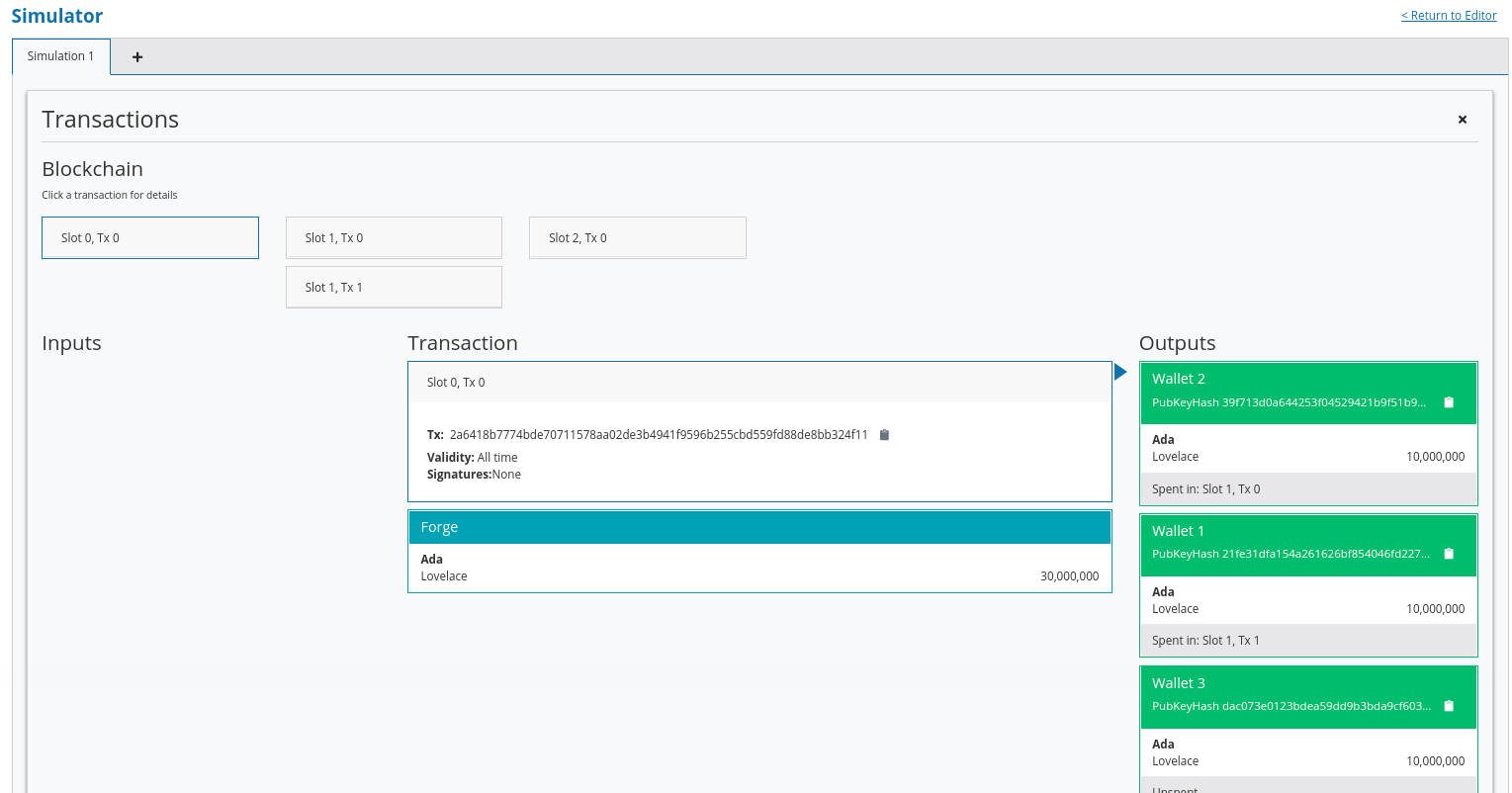And there are two transactions which occur at slot 1. They are the two `give` transactions.

The first one, Tx 0, is from wallet 2. The order here is not determined by the order that we created the transactions in the simulator. The important thing to note is that both `give` transactions occurred at the same slot.

We see the three outputs. The first output is the 10 lovelace fee paid by wallet 2. The second output is the 6 Ada sent to the script address, and the third output is the returning of the change to wallet 2, which is 4 Ada minus the fees.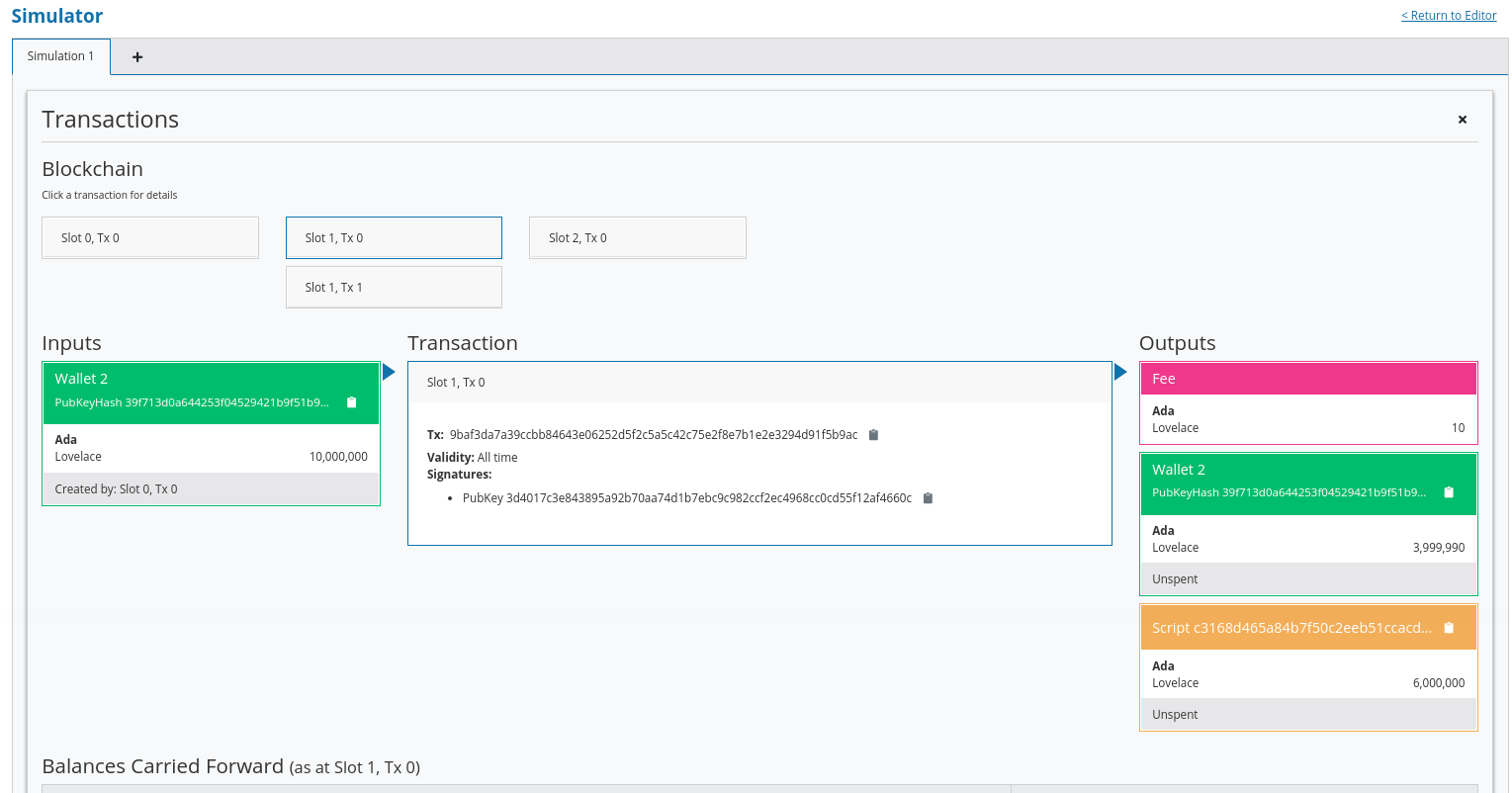And the second, Tx 1, is from wallet 1. Again, with similar output UTxOs.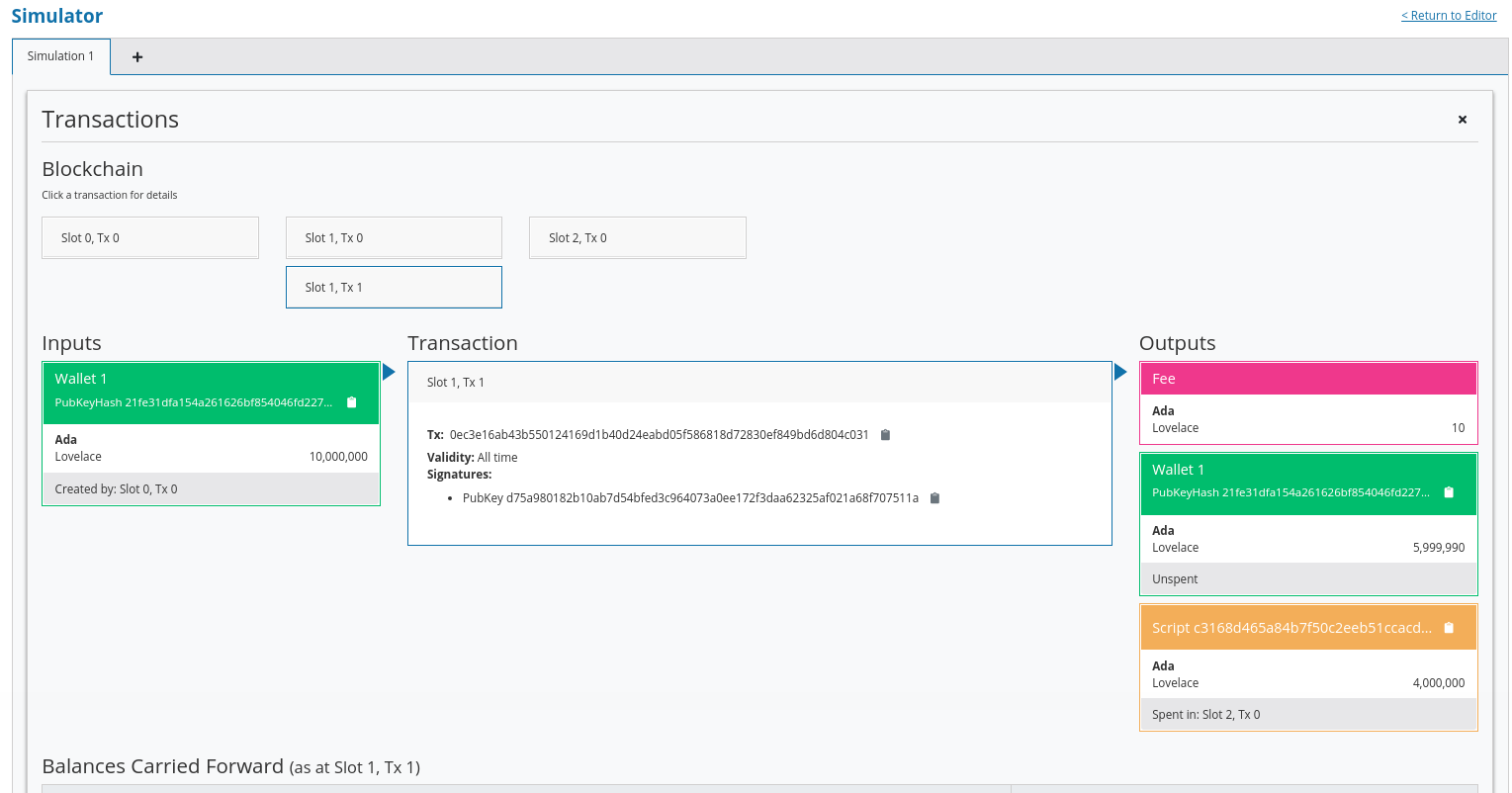We now have two UTxOs sitting at the script address.

Then we have the `grab` at slot 2 triggered by wallet 3. We see the two UTxOs from the script as inputs, and then two outputs. One output is the fees and the other is the output, paid to wallet 3, is of 10 Ada minus those fees. You’ll notice that the fees are now higher than we saw before, and this is because a script has now been executed, which makes it more expensive. However, the fees here are not yet entirely calibrated with those that would be charged on the real blockchain.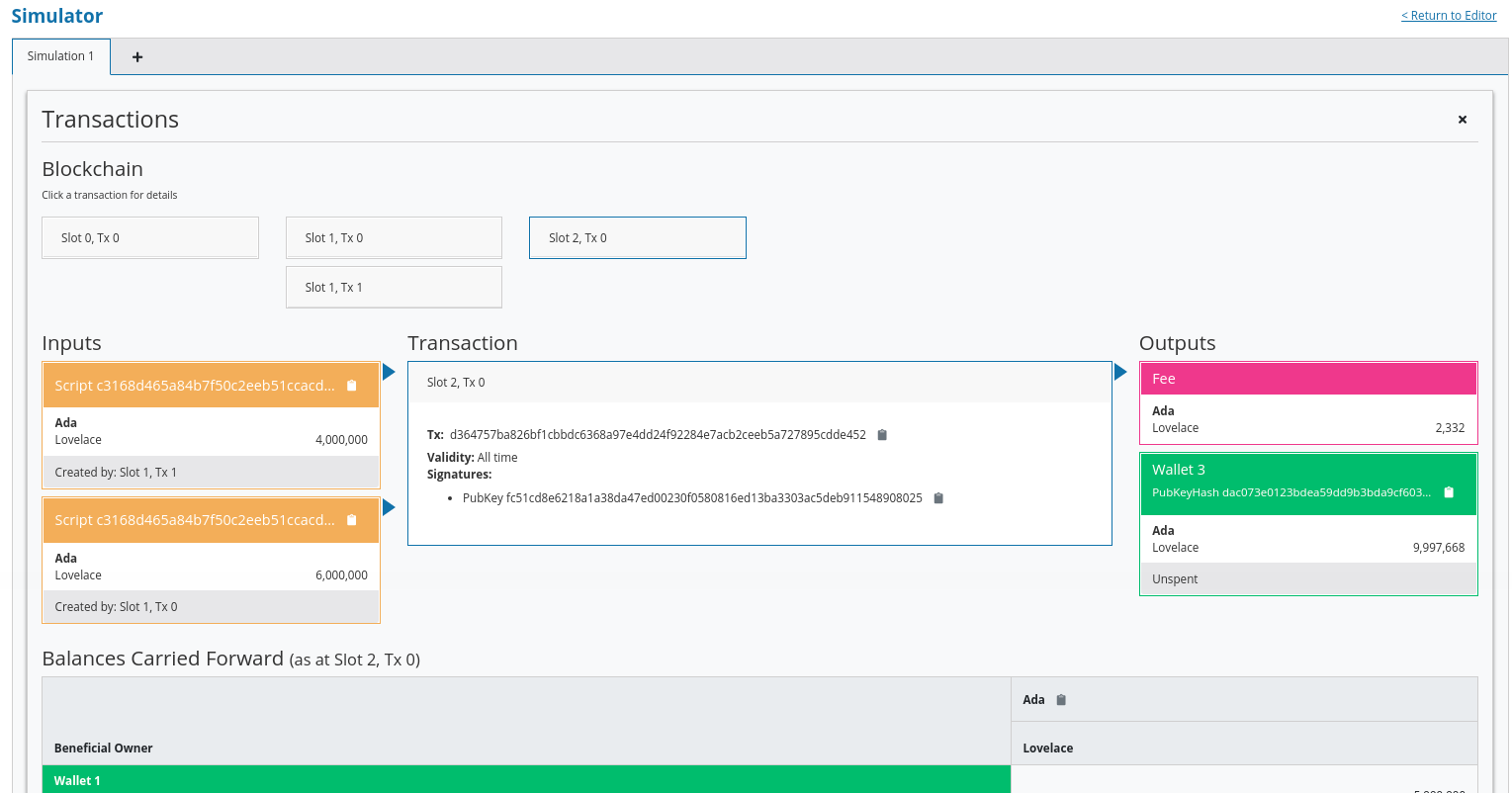And, by scrolling down, we see the final wallet balances.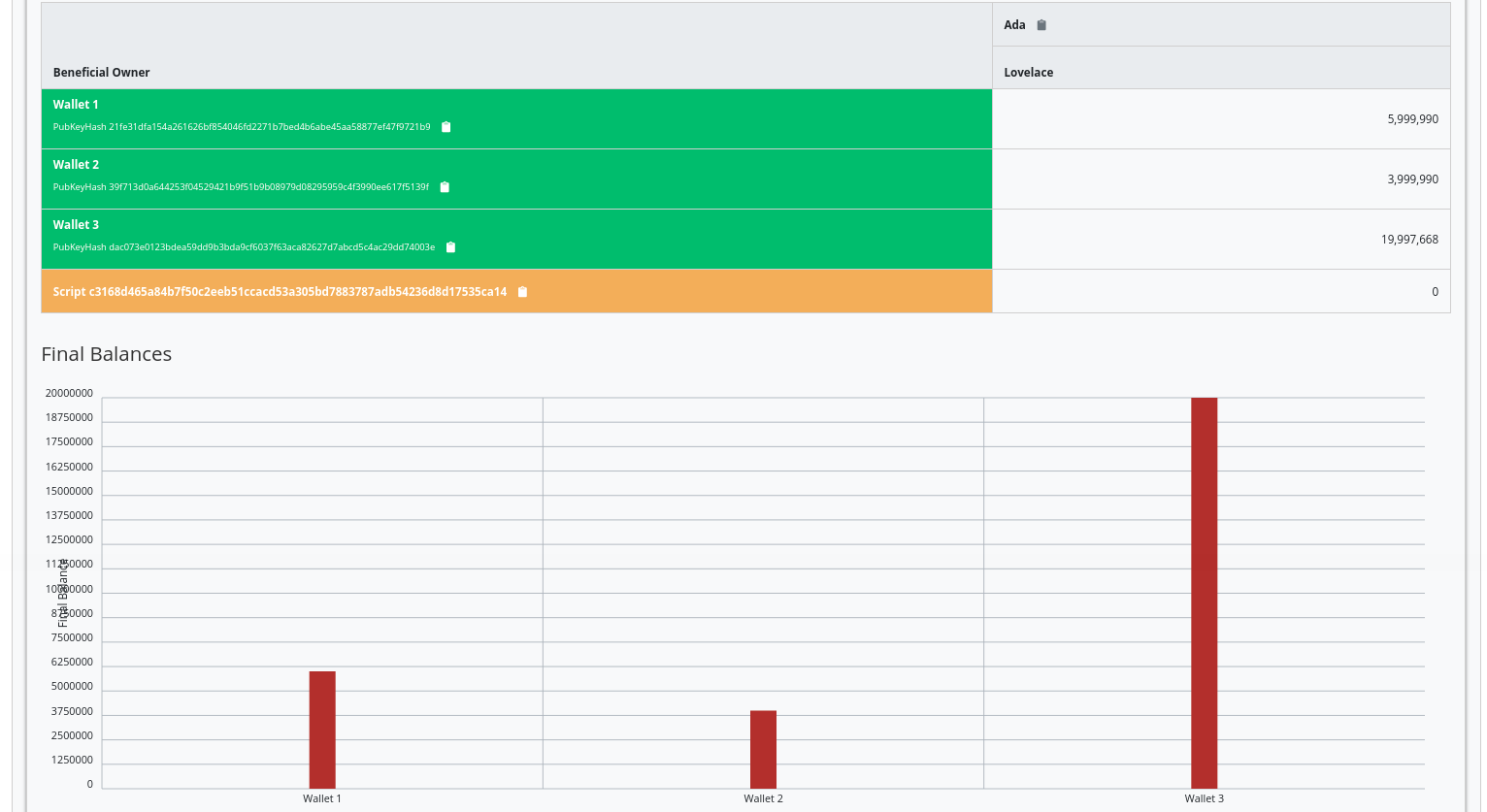If you were to scroll down further you would see some traces and log outputs that would give more detail about the execution.

As mentioned, this script uses the simplest validator possible, one that always succeeds. But this silly little validator may be useful in a situation where someone wants to donate some lovelace to the community and leave it up for grabs!

### 2.4.2. Example 2 - Burn¶

Let’s look at the second example of validation.

We will start by copying the `Gift.hs` code and renaming it `Burn.hs`.

In the `Gift` example we had a validator that would always succeed. In this example, we want to do the opposite - a validator that always fails.

Recall that a validator indicates failure by throwing an error. So we can modify our validator accordingly.

```mkValidator :: Data -> Data -> Data -> ()
mkValidator _ _ _ = error ()
```

If we load the module in the REPL and look at error

```Prelude Week02.Burn> :t error
error :: [Char] -> a
```

We see the definition for the `error` function defined in the standard Haskell `Prelude`. However, the one in scope in our code is in fact the following `error` function.

```Prelude Week02.Burn> :t PlutusTx.Prelude.error
PlutusTx.Prelude.error :: () -> a
```

In regular Haskell, you have the `error` function which takes an error message string and triggers an error. In Plutus, the `error` function does not take a string - it just takes `()` and returns an arbitrary type.

And that takes us to an important point.

We mentioned earlier that we use the `INLINABLE` pragma on the `mkValidator` function in order to allow it to be used by the Template Haskell code. In Haskell there are many functions available via the `Prelude` module, but these will not be usable in Plutus as they are not defined as inlinable. So, the Plutus team have provided an alternative Prelude that can be used in validation.

The way that the Plutus Prelude is able to take precedence over the Haskell Prelude, which is normally in scope by default, is by using the following `LANGUAGE` pragma in the code.

```{-# LANGUAGE NoImplicitPrelude   #-}
```

Then, by importing `PlutusTx.Prelude`, its functions are used in place of the standard Prelude functions.

```import PlutusTx.Prelude hiding (Semigroup(..), unless)
```

You may also notice that the standard Prelude is also imported. However, it is only in order to bring in some functions that have nothing to do with validation but is for the off-chain code and the playground.

```import Prelude (IO, Semigroup (..), String)
```

It can be confusing. A lot of the functions in the Plutus Prelude do have the same signatures and same behaviour as their namesakes in the standard Prelude, but that is not always the case, and `error` is an example.

Just remember that when you are using something in a Plutus script that looks like a function from the standard Prelude, what you are actually using is a function from the Plutus Prelude. Often they will have the same signature, but they are not always identical - for example operator precedents may not be the same

Looking again at our new validator, we now have a validator that will always fail.

```mkValidator :: Data -> Data -> Data -> ()
mkValidator _ _ _ = error ()
```

We will leave everything else as it was and check the effect of this change, using the playground. After clicking `Compile`, the previous scenario should still be present in the simulator. And after clicking `Evaluate` and scrolling down a little, we can see that wallets 1 and 2 have made their gifts but wallet 3 has been unable to grab.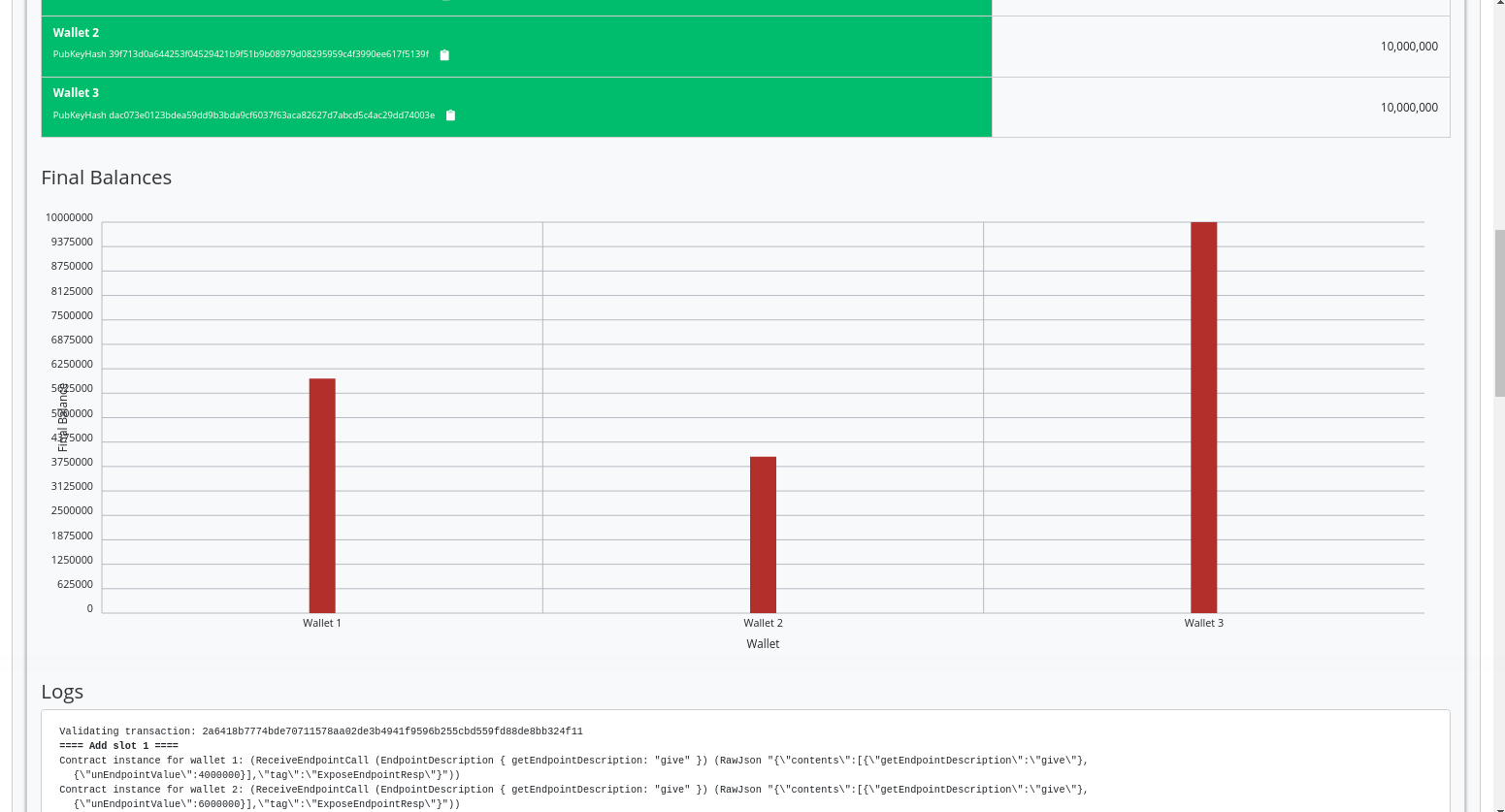If we scroll down further, we will find a log message showing that validation failed.

```, Slot 2: 00000000-0000-4000-8000-000000000002 {Contract instance for wallet 3}:
Contract instance stopped with error: "WalletError (ValidationError (ScriptFailure (EvaluationError [])))" ]
```

So, in our first example we had a validator that would always succeed and would allow anyone to grab the UTxOs from it. In the second example, we have a validator that always fails and any UTxOs sent to this script address can never be retrieved. This is basically a way to burn funds, which may be useful under some circumstances.

When we look at the logs, we see that validation fails, but we have no clue why it fails. here’s a way to change that by using a variant of error - `traceError`.

```Prelude Week02.Burn> :t PlutusTx.Prelude.traceError
PlutusTx.Prelude.traceError :: PlutusTx.Builtins.String -> a
```

The function takes a string, but not a Haskell string. It is a Plutus string. In order for this to compile, we need to use the `OverloadedStrings` GHC extension.

```{-# LANGUAGE OverloadedStrings   #-}
```

Then, we can update our validator.

```mkValidator _ _ _ = traceError "BURNT!"
```

If we now run the same scenario in the playground with the new code, we will see the custom error message that we added.

```, Slot 2: 00000000-0000-4000-8000-000000000002 {Contract instance for wallet 3}:
Contract instance stopped with error: "WalletError (ValidationError (ScriptFailure (EvaluationError [\"BURNT!\"])))" ]
```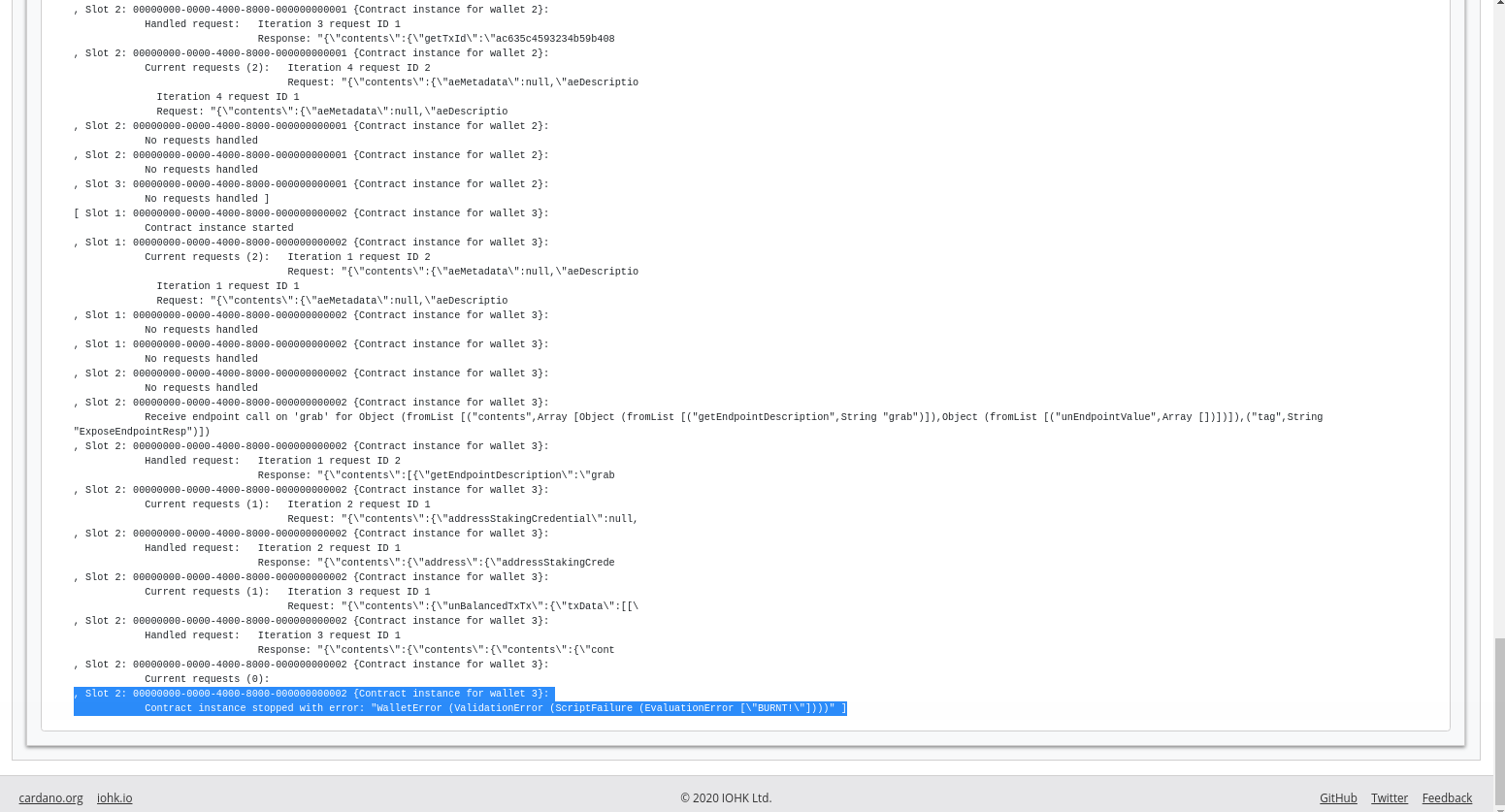### 2.4.3. Example 3 - Forty Two¶

For the next example, we will write a validator that does not completely ignore all its arguments. We’ll write one that expects a simple redeemer.

Now that we care about the redeemer, we need to be able to reference it. Let’s call it `r`.

```{-# INLINABLE mkValidator #-}
mkValidator :: Data -> Data -> Data -> ()
mkValidator _ r _
```

Let’s say that we want validation to pass if the redeemer is `I 42`.

```{-# INLINABLE mkValidator #-}
mkValidator :: Data -> Data -> Data -> ()
mkValidator _ r _
| r == I 42 = ()
| otherwise = traceError "wrong redeemer"
```

If we were to run this now in the playground, validation would always fail. We need to modify the off-chain code to add an input to the `grab` endpoint so that wallet 3 can pass in an `Integer` which we can then pass to the validator as the redeemer.

```type GiftSchema =
Endpoint "give" Integer
.\/ Endpoint "grab" Integer
```

We add the redeemer argument to the `grab` declaration. Note the addition of the `Integer` in the function signature, as well as the new `n` parameter which is used to reference it.

```grab :: forall w s e. AsContractError e => Integer -> Contract w s e ()
grab n = do
```

We can then pass it to the `mustSpendScriptOutput` function instead of the throw-away value we used earlier.

```tx = mconcat [mustSpendScriptOutput oref \$ Redeemer \$ I n | oref <- orefs]
```

One more change, we need to change the `>>` to `>>=` in the following code, now that `grab` has an argument. You can use the REPL to look at the types `>>` and `>>=` to see why the second one is now needed. Basically, they both sequence actions, but `>>` ignores any wrapped values, whereas `>>=` accesses the wrapped value and passes it to the next action.

```grab' = endpoint @"grab" >>= grab
```

Now we can try it out in the playground. After adding the new code and clicking `Simulate` you will notice that the old scenario has gone. That is because the endpoints have changed and the old scenario is no longer valid.

Let’s set up a scenario that uses just two wallets. Wallet one is going to give 3 Ada oo the contract, and wallet 2 is going to try to grab them, but this time, wallet 2 will need to pass in a value which will be used to construct the redeemer.

For our first attempt, we will add the wrong redeemer value, in this case 100.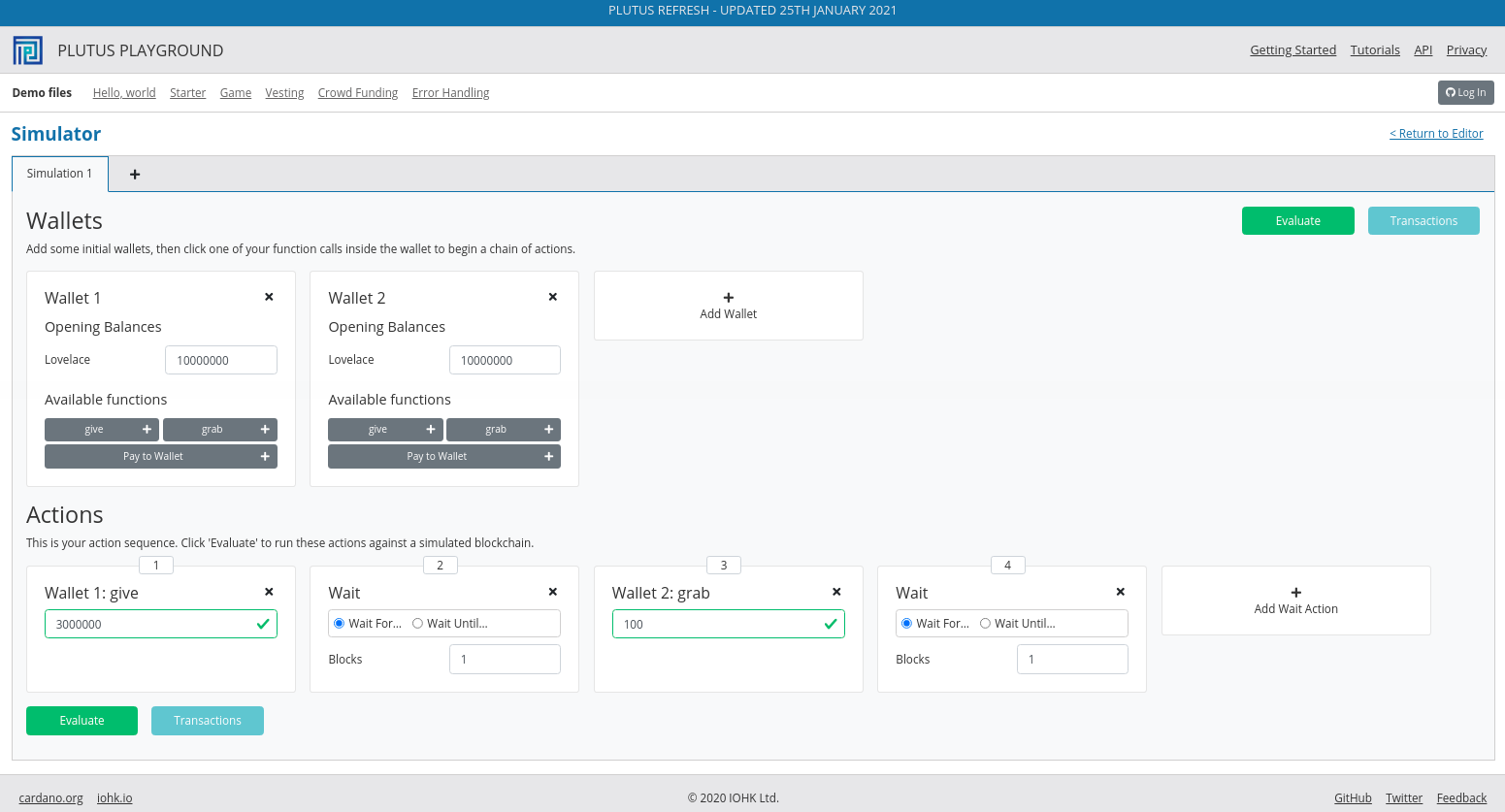If we click `Evaluate`, we see that we only have two transactions, and we see that the Ada remains in the script, which shows that wallet 2 failed to grab it.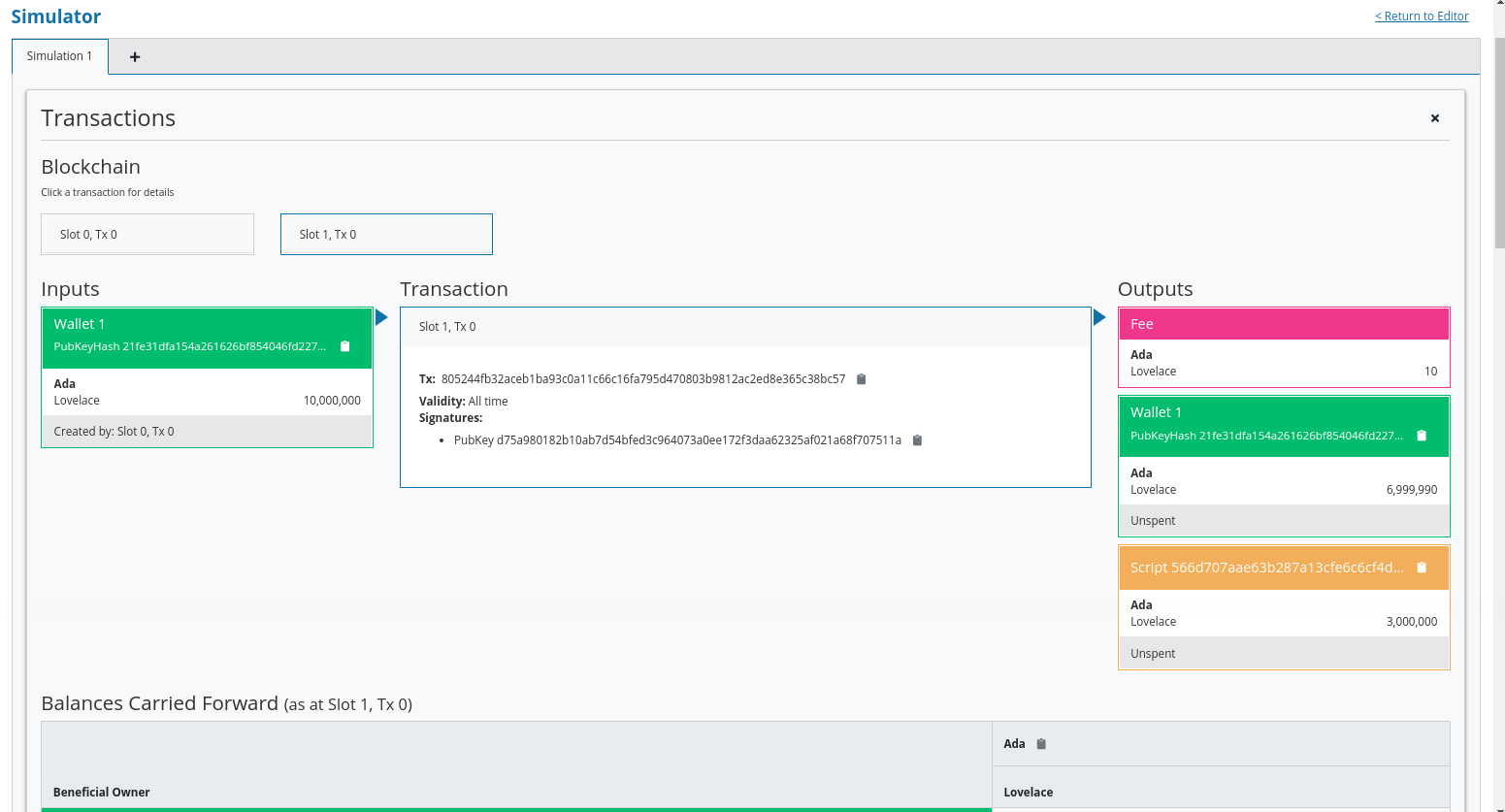The final balances also show this.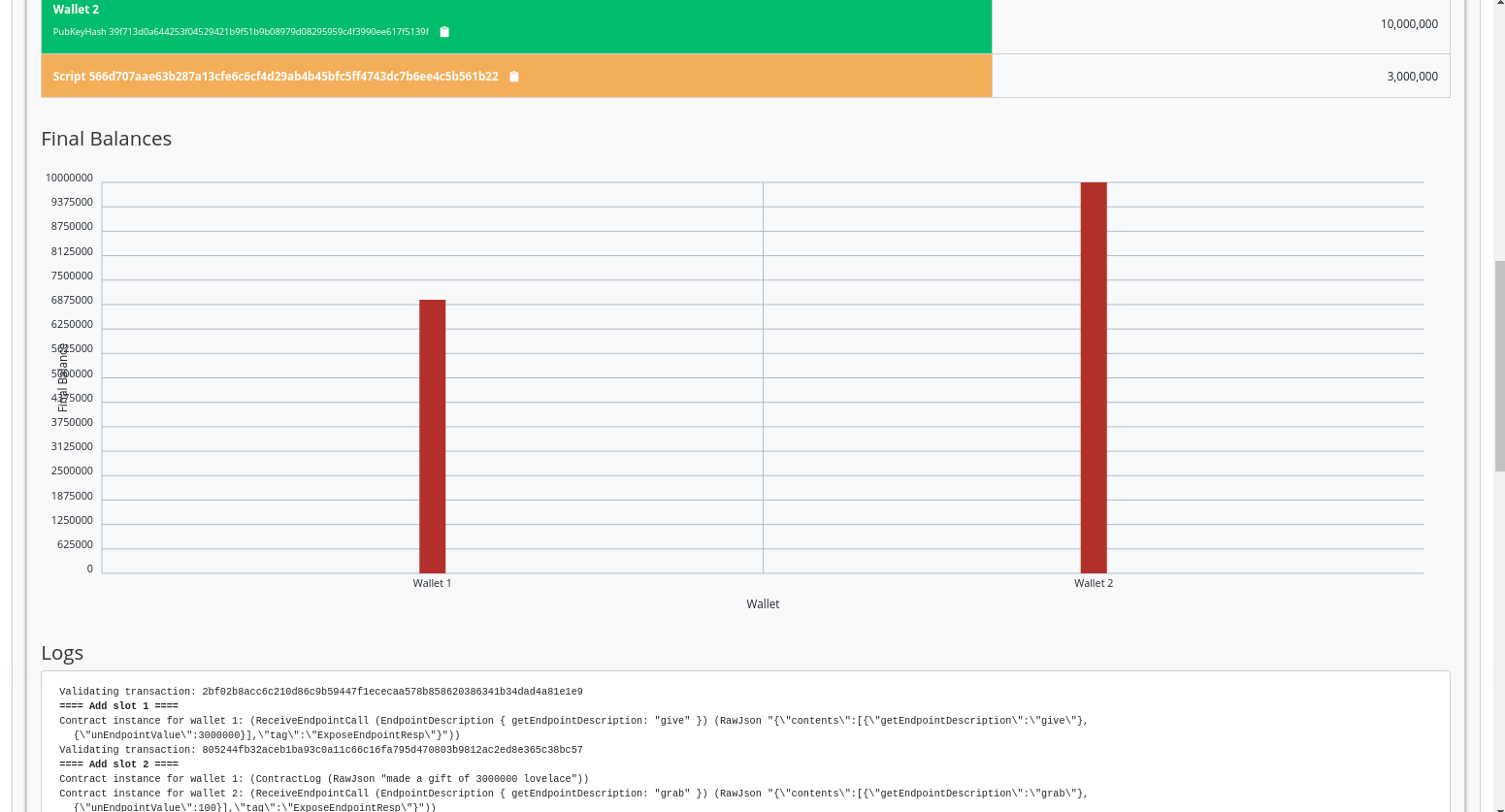And, if we look at the trace, we find the error.

```, Slot 2: 00000000-0000-4000-8000-000000000001 {Contract instance for wallet 2}:
Contract instance stopped with error: "WalletError (ValidationError (ScriptFailure (EvaluationError [\"wrong redeemer\"])))" ]
```

If we go back to scenario, change the value to `42` and click `Evaluate` again, we should see that validation succeeds.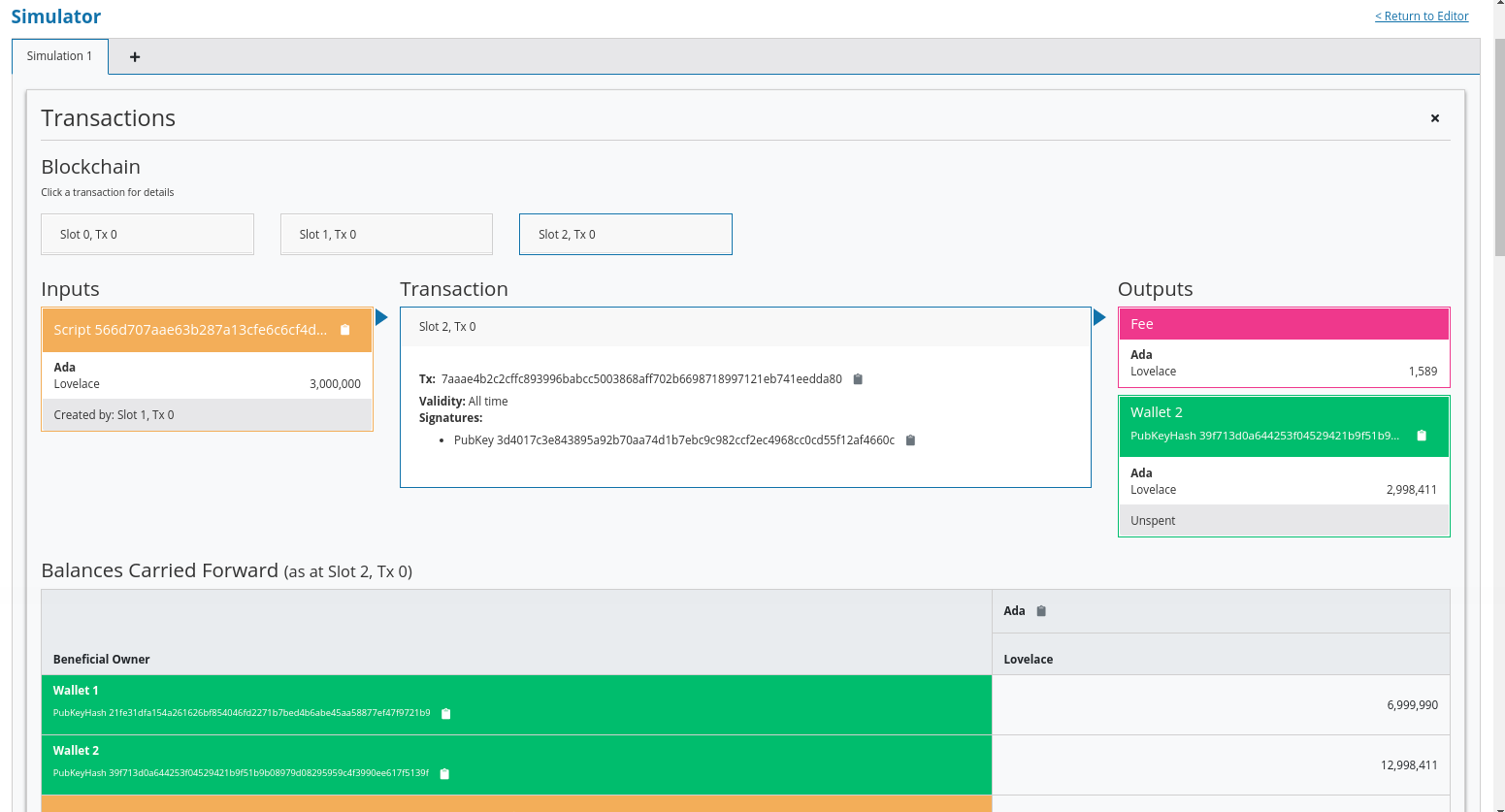Now we see the third transaction where wallet 2 manages to collect the funds, minus fees.We see that the final balances are as we expect, and also the logs show that validation did not throw an error, which means that validation succeeded.

So that’s the first example of a validator that looks at at least one of its arguments.

### 2.4.4. Example 4 - Typed¶

It was mentioned at the beginning of the lecture, this is low-level Plutus and in reality, no-one will write validation functions like this.

Now we will see how it is actually done using a typed version.

Even though the `Data` type is powerful and you can encode all sorts of data into it, it doesn’t really feel like Haskell. It is almost like you are writing in an untyped language like Javascript or Python. It is just a like a blob of data, it can contain anything so you don’t really have any type safety. You will always need to check, for example, if you are expecting an integer that you are indeed given an integer.

It is especially bad with the third argument, the context. Even though it’s easy to imagine that you can somehow encode a transaction with its inputs and outputs into the `Data` type, it is not at all clear how that is done.

We would rather use more specific data types that are tailored to the business logic.

This is indeed possible with so-called Typed Validators. What this means is that we can replace the occurrences of `Data` in the `mkValidator` signature with more suitable types.

```mkValidator :: Data -> Data -> Data -> ()
```

In our silly little example, we completely ignore the Datum, so a more suitable type would be just the Unit type - ().

```mkValidator :: () -> Data -> Data -> ()
```

For the redeemer, in this example, we are only dealing with integers, so it would probably make more sense to use Integer instead.

```mkValidator :: () -> Integer -> Data -> ()
```

For the context, there is a much nicer type called `ScriptContext` that’s made exactly for this purpose.

```mkValidator :: () -> Integer -> ScriptContext -> ()
```

Finally, we have already mentioned that it is a bit unusual to use `()` as a return type. Much more natural would be to use `Bool` to indicate successful or failed validation.

```mkValidator :: () -> Integer -> ScriptContext -> Bool
```

So, this is a better way to write validation code. The last two types `SciprtContext` and `Bool`, but the first two types can be different depending on the situation.

In this case, let’s now rewrite the function accordingly using these new types. The parameter `r` is now no longer of type `Data` - it is an `Integer`, so we can simply check that it is equal to 42 rather than checking it against a constructed `Data` type.

And, as we are now returning a `Bool`, we can we just make the function a boolean expression.

```{-# INLINABLE mkValidator #-}
mkValidator :: () -> Integer -> ScriptContext -> Bool
mkValidator _ r _ = r == 42
```

This will have the same problem that we had before in that, in the case of an error, we won’t get a nice error message. There is a nice Plutus function called `traceIfFalse` which takes a `String` and a `Bool` and returns a `Bool`. If the first `Bool` is `True`, the result will be `True` and the `String` is ignored. However, if the first `Bool` is `False`, then the result will be `False` and the `String` will be logged.

```PlutusTx.Prelude.traceIfFalse
:: PlutusTx.Builtins.String -> Bool -> Bool
```

This is exactly what we need.

```{-# INLINABLE mkValidator #-}
mkValidator :: () -> Integer -> ScriptContext -> Bool
mkValidator _ r _ = traceIfFalse "wrong redeemer" \$ r == 42
```

This will not yet compile as other parts of the code are not yet type correct. We need to adapt our boilerplate.

First, we introduce a new dummy data type, which here we call `Typed`, simply based on the name of the script. For this type we must provide an instance of `Scripts.ValidatorTypes`.

The purpose of this instance is to declare the types for the datum and the redeemer.

```data Typed
instance Scripts.ValidatorTypes Typed where
type instance DatumType Typed = ()
type instance RedeemerType Typed = Integer
```

This is quite advanced Haskell, so-called type-level programming, but just like the Template Haskell we have already encountered, you don’t really need a deep understanding of it as all scripts will follow the same pattern.

Now we need to compile the validator. Where previously we used `mkValidatorScript`, now we use something called `mkTypedValidator`, which takes our new data type as parameter and produces something of type `TypedValidator`.

```typedValidator :: Scripts.TypedValidator Typed
typedValidator = Scripts.mkTypedValidator @Typed
\$\$(PlutusTx.compile [|| mkValidator ||])
\$\$(PlutusTx.compile [|| wrap ||])
where
wrap = Scripts.wrapValidator @() @Integer
```

This is similar to the `mkValidator` code, but this type we also compile a `wrapValidator` function that takes the datum and redeemer types.

In order for this to work we first need one more import.

```import qualified Ledger.Typed.Scripts as Scripts
```

In this example, it is being imported qualified and using the `Scripts` prefix, but this is arbitrary and you could pick some other way of referencing the module.

We these changes, the Haskell code will compile, and we now need to change the Template Haskell boilerplate that creates the `validator` function.

```validator :: Validator
validator = Scripts.validatorScript typedValidator
```

Here we have used the `validatorScript` function to create an untyped validator from our typed version.

To get the hash we could, of course, use the validator we now have and turn it into a `ValidatorHash` as we did before, but there is a more direct way, which looks identical, but in this case `Scripts` is coming from the module `Ledger.Typed.Scripts` rather than `Ledger.Scripts`. This version takes the typed validator directly.

```valHash :: Ledger.ValidatorHash
valHash = Scripts.validatorHash typedValidator
```

The script address is calculated as before.

```scrAddress :: Ledger.Address
```

In this extremely simply example, it probably doesn’t seem worth the effort, but for realistic contracts, it is much nicer to do it like this.

The off-chain code is almost identical.

There is a small change change to the `give` endpoint. Although we have not yet gone over this part of the code in detail, the following changes can be made.

```let tx = mustPayToTheScript () \$ Ada.lovelaceValueOf amount
ledgerTx <- submitTxConstraints inst tx
```

The `mustPayToOtherScript` function has been replaced with `mustPayToTheScript`. This is a convenience script which allows us to pass in just () as we longer need to construct a value of type `Data`. We also no longer need to pass in the script hash.

The behaviour of this code will be identical to the behaviour in the previous example, so we won’t go over it in the playground.

Now we will explain how that actually works. How does Plutus convert these custom data types to the actual low-level implementation - the `Data` type.

We can look at the code in the `PlutusTx.IsData.Class` module.

Here we see that there is a quite simple type class defined called `IsData`.This class provides two functions

• `toData` takes a value and converts it to `Data`

• `fromData` takes a value of type `Data` and attempts to convert it to an instance of type `IsData`. This can fail because not all values of type `Data` will be convertible to the target type.

Let’s try this out in the REPL.

```Prelude Week02.Typed> import PlutusTx
Prelude PlutusTx Week02.Typed> import PlutusTx.IsData.Class
Prelude PlutusTx PlutusTx.IsData.Class Week02.Typed> :i IsData
```

We know that `()` and `Integer` are both instances of `IsData` because they worked in our example.

Let’s convert an `Integer` to `Data`

```Prelude PlutusTx PlutusTx.IsData.Class Week02.Typed> toData (42 :: Integer)
I 42
```

We see that this has been converted to an instance of type `Data` using the `I` constructor, which we did manually before we used typed validation.

Now let’s do it the other way around

```Prelude PlutusTx PlutusTx.IsData.Class Week02.Typed> fromData (I 42) :: Maybe Integer
Just 42
```

We get a `Just 42` back - `Just` being the `Maybe` constructor when `Maybe` is not `Nothing`.

And when it fails, when it can’t convert to the target type, we will get back `Nothing`.

```Prelude PlutusTx PlutusTx.IsData.Class Week02.Typed> fromData (List []) :: Maybe Integer
Nothing
```

If we examine `IsData` we can see all the types that this pattern will work for all the types that have an `IsData` instance defined.

```Prelude PlutusTx PlutusTx.IsData.Class Week02.Typed> :i IsData
type IsData :: * -> Constraint
class IsData a where
toData :: a -> Data
fromData :: Data -> Maybe a
{-# MINIMAL toData, fromData #-}
-- Defined in ‘PlutusTx.IsData.Class’
instance IsData a => IsData (Maybe a)
-- Defined in ‘plutus-tx-0.1.0.0:PlutusTx.IsData.Instances’
instance (IsData a, IsData b) => IsData (Either a b)
-- Defined in ‘plutus-tx-0.1.0.0:PlutusTx.IsData.Instances’
instance IsData Bool
-- Defined in ‘plutus-tx-0.1.0.0:PlutusTx.IsData.Instances’
instance (IsData a, IsData b, IsData c, IsData d) =>
IsData (a, b, c, d)
-- Defined in ‘plutus-tx-0.1.0.0:PlutusTx.IsData.Instances’
instance (IsData a, IsData b, IsData c) => IsData (a, b, c)
-- Defined in ‘plutus-tx-0.1.0.0:PlutusTx.IsData.Instances’
instance (IsData a, IsData b) => IsData (a, b)
-- Defined in ‘plutus-tx-0.1.0.0:PlutusTx.IsData.Instances’
instance IsData ()
-- Defined in ‘plutus-tx-0.1.0.0:PlutusTx.IsData.Instances’
instance IsData a => IsData [a]
-- Defined in ‘PlutusTx.IsData.Class’
instance IsData Integer -- Defined in ‘PlutusTx.IsData.Class’
instance (TypeError ...) => IsData Int
-- Defined in ‘PlutusTx.IsData.Class’
instance IsData Data -- Defined in ‘PlutusTx.IsData.Class’
```

This is still quite a short list of possible types. We would like to use many more types than this for our datum and redeemer.

In order to do this, we would normally need to define an `IsData` instance for any type that we wish to use. This will allow us to tell the compiler how to do the back and forth conversions. However, this again would be tedious as it is such a mechanical process. So, there is a mechanism in Plutus that does this for us.

### 2.4.5. Example 5 - Custom IsData types¶

Now let’s talk about custom data types. Let’s define a silly one and use it in our validator function.

```newtype MySillyRedeemer = MySillyRedeemer Integer

PlutusTx.unstableMakeIsData ''MySillyRedeemer

{-# INLINABLE mkValidator #-}
mkValidator :: () -> MySillyRedeemer -> ScriptContext -> Bool
mkValidator () (MySillyRedeemer r) _ = traceIfFalse "wrong redeemer" \$ r == 42
```

Note

There is also a stable version of the `PlutusTx.unstableMakeIsData` function, and the stable version should always be used in production code. The difference between the two is that, in the case where more than one `Data` constructor is required, the unstable version makes no guarantee, between Plutus versions, that the order of constructors will be preserved.

And we need to change some of the boilerplate.

```data Typed
instance Scripts.ValidatorTypes Typed where
...
type instance RedeemerType Typed = MySillyRedeemer

typedValidator :: Scripts.TypedValidator Typed
...
where
wrap = Scripts.wrapValidator @() @MySillyRedeemer
```

We also need to change some off-chain code in the `grab` endpoint.

Instead of using `I r`, we will use `toData (MySillyRedeemer r)`.

```grab :: forall w s e. AsContractError e => Integer -> Contract w s e ()
grab r = do
let orefs   = fst <\$> Map.toList utxos
lookups = Constraints.unspentOutputs utxos      <>
Constraints.otherScript validator
tx :: TxConstraints Void Void
tx      = mconcat [mustSpendScriptOutput oref \$ Redeemer \$ PlutusTx.toData (MySillyRedeemer r) | oref <- orefs]
ledgerTx <- submitTxConstraintsWith @Void lookups tx
void \$ awaitTxConfirmed \$ txId ledgerTx
```

If we try to compile the code now, either on the command line or in the playground, we will get an error because Plutus doesn’t know how to convert back and forth between `IsData` and `MySillyRedeemer`.

We could write an instance of `IsData` for `MySillyRedeemer` by hand. But, we don’t need to.

```PlutusTx.unstableMakeIsData ''MySillyRedeemer
```

At compile time, the compiler will use the Template Haskell to write an `IsData` instance for us. And now, it will compile.

Let’s check it in the REPL.

```Prelude PlutusTx PlutusTx.IsData.Class> :l src/Week02/IsData.hs
Prelude PlutusTx PlutusTx.IsData.Class Week02.IsData> toData (MySillyRedeemer 42)
Constr 0 [I 42]
```

If you try this code, which is in `IsData.hs`, in the playground, you should see that it behaves in the same way as before.

## 2.5. Summary¶

We have seen a couple of examples of simple validators.

We started with a validator that will always succeed, completely ignoring its arguments. Then we looked at a validator that always fails, again completely ignoring its arguments. Then we looked at one that examines its redeemer to check for a certain predefined value.

We then turned this validator into a typed version which is the one which would be used in practice. First we used built-in data types and then we saw how we can use custom data types.

We have not yet looked at examples where the datum or the context are inspected, which would be required for more realistic examples.

We will look at that in the next lecture.